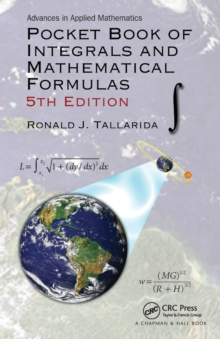Supporting your high street Find out how »
• My AccountPocket Book of Integrals and Mathematical Formulas Paperback / softback

Description

Pocket Book of Integrals and Mathematical Formulas, 5th Edition covers topics ranging from precalculus to vector analysis and from Fourier series to statistics, presenting numerous worked examples to demonstrate the application of the formulas and methods.

This popular pocket book is an essential source for students of calculus and higher mathematics courses.

It also provides an easy-to-use, accessible reference for mathematicians, engineers, scientists, and others seeking vital mathematical formulas, concepts, and definitions.

Enlarging the type without sacrificing special topics involving financial mathematics and number theory, this 5th Edition:Includes several classic calculus applications that illustrate the power and practical use of calculusDiscusses an interesting offshoot of Fermat's last theorem, namely, "near misses"Reformats and revises the table of integrals for improved clarity and accuracyThrough careful selection of topics and detail, Pocket Book of Integrals and Mathematical Formulas, 5th Edition remains a portable yet comprehensive resource for students and professionals, containing the most important mathematical formulas for engineering and scientific applications.

Information

• Format: Paperback / softback
• Pages: 387 pages, 17 Tables, black and white; 61 Illustrations, black and white
• Publisher: Taylor & Francis Inc
• Publication Date:
• Category: Calculus & mathematical analysis
• ISBN: 9781498704755

£25.99

£23.69

on all orders

Pick up orders

from local bookshops